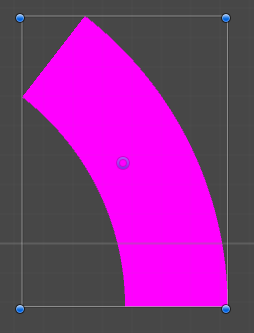# How can I draw this 2d shape? (two straight lines and two curved segments)

I only need to draw two of them, so the method doesn’t need to be particularly fast.
I just don’t know where to start. Are there any smallish libraries that can help me accomplish this?Do you need to do it in unity, or can a program like, say, inkscape make it, and import it. A much easier method, if that is all you need to do.Quickly made a script to do this for
you. Obviously you don’t want to be
generating the mesh every frame so
just use it to generate it once then
draw it however you want.``````using UnityEngine;
using System.Collections;

[RequireComponent(typeof(MeshRenderer), typeof(MeshFilter)), ExecuteInEditMode]
public class CircleMesh : MonoBehaviour
{
public int SegmentCount;
public float DeltaStart;
public float DeltaSize;

private Mesh _mesh;

private void Awake()
{
_mesh = new Mesh();

GetComponent<MeshFilter>().sharedMesh = _mesh;
}

private void Update()
{
UpdateMesh();
}

private void UpdateMesh()
{
_mesh.Clear();

Vector3[] vertices = new Vector3[(SegmentCount + 1) * 2];
int[] indices = new int[SegmentCount * 6];

for (int i = 0; i <= SegmentCount; ++i)
{
float angle = (DeltaStart * Mathf.Deg2Rad) + ((DeltaSize * Mathf.Deg2Rad) / SegmentCount) * i;
Vector2 direction = new Vector2(Mathf.Cos(angle), Mathf.Sin(angle));

vertices[i * 2] = direction * OuterRadius;
vertices[(i * 2) + 1] = direction * InnerRadius;
}

for (int i = 0; i < SegmentCount; ++i)
{
int baseIndex = i * 6;

indices[baseIndex] = (i * 2);
indices[baseIndex + 1] = ((i * 2) + 1);
indices[baseIndex + 2] = (((i + 1) * 2) + 1);

indices[baseIndex + 3] = (((i + 1) * 2) + 1);
indices[baseIndex + 4] = ((i + 1) * 2);
indices[baseIndex + 5] = (i * 2);
}

_mesh.vertices = vertices;
_mesh.triangles = indices;
}
}
``````